lite-C pointers
 This month's "Plug and play" article is in fact a workshop that discusses one of the most misunderstood programming chapters: lite-C pointers. There are lots of beginners who don't know how and when to use pointers, so we'll (hopefully) deal with them for good. We will have several "plug and play" pointer examples, of course. Oh, and don't forget to check out the workshop from Aum70 if you'd like to learn more about standard C pointers.   As you might know, any variable has to reside somewhere in the computer's memory, which is split into individual cells that can hold values. Let's say that I have defined a bunch of variables like this:   int shotgun_bullets = 12; var players_health = 100; double seconds_passed = 1000000;   These are all valid variable definitions; if we include them in a lite-C script and we run it, we will know for sure that we've got an integer variable named shotgun_bullets that has a value of 12 and sits somewhere in one or more memory cells, and so on. Our computer memory could look like this: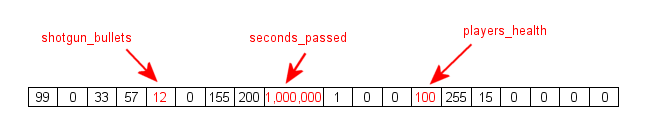Nobody will be able to tell you where your variables have ended up in the memory, but one thing is for sure: they will occupy one or more memory cells. Now wouldn't it be nice to actually know how many memory cells (how many bytes) are used by each one of our variables?   The lite-C language has a built-in a powerful function named sizeof( ) which does just that: it tells us the size (in bytes) that's occupied by a variable or by a struct in memory. Fire up Sed, and then open and run pointers1.c; you will see the following image: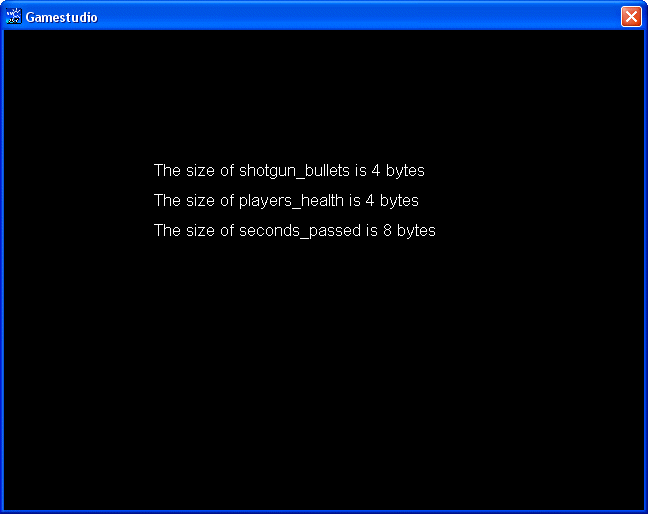Let's take a look at the code that makes it happen:   #include #include   int shotgun_bullets = 12; var players_health = 100; double seconds_passed = 1000000; var int_size, var_size, double_size;   FONT* arial_font = "Arial#20";   PANEL* time_pan = {        layer = 15;        digits (150, 130, "The size of shotgun_bullets is %.f bytes", arial_font, 1, int_size);                digits (150, 160, "The size of players_health is %.f bytes", arial_font, 1, var_size);                digits (150, 190, "The size of seconds_passed is %.f bytes", arial_font, 1, double_size);                flags = visible; }   function main() {        video_mode = 6; // create a program window of 640x480 pixels        vec_set(screen_color, vector(0, 0, 0)); // make the background color black        int_size = sizeof(shotgun_bullets);        var_size = sizeof(players_health);        double_size = sizeof(seconds_passed); }   There's nothing complicated here; function main does the entire job. We have defined 3 variables named int_size, var_size, double_size and we use sizeof(variable_name) to display their values using 3 "digits" instructions and a panel.   Ok, so now we know how many bytes are reserved for our variables; in fact, if you search the manual you will find a table that gives us this information. But wouldn't it be nice if we would be able to access those memory cells that store our variables directly? This would also allow us to access complex data structures (arrays, matrices, etc) easily, to reserve some memory areas for our own use, and so on.   Let's open and run pointers2.c: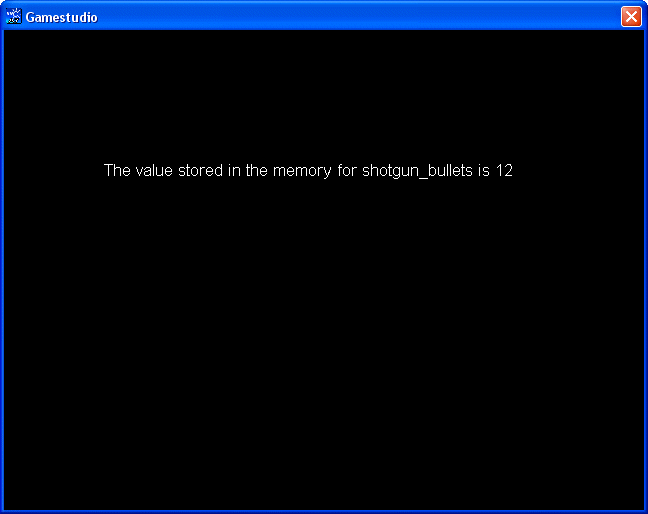#include #include   int shotgun_bullets = 12; var cell_value;   FONT* arial_font = "Arial#20";   PANEL* time_pan = {        layer = 15;        digits (100, 130, "The value stored in the memory for shotgun_bullets is %.f", arial_font, 1, cell_value);        flags = visible; }   function main() {        video_mode = 6; // create a program window of 640x480 pixels        vec_set(screen_color, vector(0, 0, 0)); // make the background color black        int *p;        p = &shotgun_bullets;        cell_value = *p; }   Function main contains three lines of code that capture the essence of working with pointers; if you understand these lines, you have won 90% of the battle. There we go:   1) int *p; declares a pointer, a variable that can store a memory address. We don't use pointers to store the values of our variables, but to store the addresses of the memory cells where the variables are placed.   We can define all sorts of pointers; these are all valid pointer definitions:   int *my_pointer; // pointer to an integer variable float *my_new_pointer; // pointer to a float variable char *my_character_pointer; // pointer to a character   Just like with variables, we can even define several pointers of the same type using a single line like this:   long *first_long_pointer, *second_long_pointer, *third_long_pointer;   A pointer is similar in many ways with the address of a house in your city. If you want to deliver a pizza to (or to borrow something from) a certain house, you need to know its address. In a similar way, if you want to deliver a value to a specific memory cell or to read a value that's been placed there, you need to use a pointer to that address.   2) p = &shotgun_bullets; assigns the address (&) of our variable named shotgun_bullets to p (notice that we are using p, and not *p here!). From now on, p is just like a pizza delivery guy who has learned the address of shotgun_bullets, so it can go there anytime in order to deliver pizza, get the money for it, and so on.   Ok, so this line of code has created the connection between our pointer p and the memory address that will be watched by it. Quick test: if we would display the value of p on the screen, what would we see? Take your time to think about it; meanwhile, I'm placing a picture drawn by one of the artists I've been working with here, so that you don't peek "accidentally" at the answer below...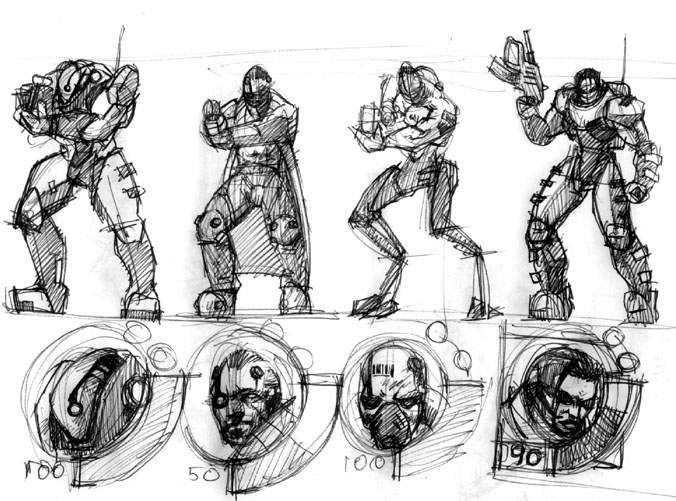The answer is simple: p would display the address of shotgun_bullets, the memory address that stores that variable. If you thought that p would be set to 12, remember that pointers don't store values, but memory addresses. We can't predict the value of the memory address pointed by p; it will change from one PC to the other, and can change even on the same PC from one program run to the other. It is comforting though to know that no matter what happens, we can keep track of our precious data using pointers - they will always point to the proper address, even if the address changes from one computer to the other, etc.   3) The third line of code is this: cell_value = *p;. This line gets the value of the memory location pointed by p and stores it inside cell_value. We know that p stores the address of shotgun_bullets (&shotgun_bullets); the asterisk operator * gives us the value that is stored at that address (the value of shotgun_bullets), which is 12. We have displayed cell_value using a "digits" instruction on a panel.   Let's review the process one more time:   - First of all, we need to define a pointer. We do this by giving it a type and a name. If we plan to point to an integer we need to use an int pointer, and so on. The name of the pointer is preceded by the asterisk operator; otherwise, the engine will think that we have defined a regular variable and not a pointer. Example: float *pointer_to_float; - If we have defined a pointer, we can assign it the address of a variable using the "&" operator. Example: pointer_to_float = &my_float_variable; - Finally, if we need to get or set the value from the memory address that's watched by our pointer, we can use the asterisk operator *. Example: test = *pointer_to_float; This process is called dereferencing; we are referring to the value pointed by the pointer. Feel free to ignore the previous phrase; it's just tech talk.   I don't know about you, but I feel that the fog starts to clear up a bit. Let's apply what we have learned about the standard C language pointers to lite-C.   Rule no. 1: all the engine objects are pointers. Take a good look at all the definitions below:   BMAP* picture1_pcx = "picture1.pcx";   SOUND* effect2_wav = "effect2.wav";   STRING* hello_str = "Hello World!";   FONT* arial_font = "Arial#20";   PANEL* gameover_pan = {        layer = 15;        pos_x = 300;        pos_y = 200;        bmap = picture1_pcx; }   TEXT* message_txt = {        pos_x = 200;        pos_y = 20;        string(hello_str);        flags = VISIBLE; }   All of these are pointer definitions and that's how you are supposed to define them. Don't use something like this because it won't work:   STRING hello_str = "Hello World!"; // this is not a pointer definition, so it won't work!   What about vectors? When and how are we supposed to use a vector pointer - VECTOR* - and when are we going to use a simple VECTOR definition? Open up pointers3.c and then run it; you will see a simple, black screen: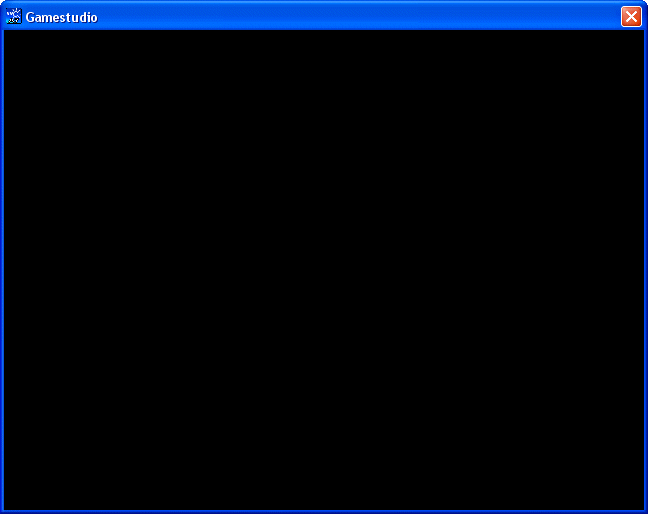Now let's take a look at the source code:   #include #include   VECTOR* my_vector;   function main() {        video_mode = 6; // create a program window of 640x480 pixels        vec_set(screen_color, vector(0, 0, 0)); // make the background color black }   This must be one of the shortest code snippets ever! The only important line of code is the one that defines the vector pointer. A vector is just a name for 3 grouped variables; you have dealt with vectors when you have used player.x, player.y, player.z and so on.   Ok, let's move forward and add another line of code; we are going to initialize the first component of my_vector. Add this line to function main:          my_vector.x = 1;   Function main( ) should look like this now:   function main() {        video_mode = 6; // create a program window of 640x480 pixels        vec_set(screen_color, vector(0, 0, 0)); // make the background color black        my_vector.x = 1; }   Save the script and run it; even though our new line of code doesn't look too dangerous, you will be greeted by an ugly engine error message.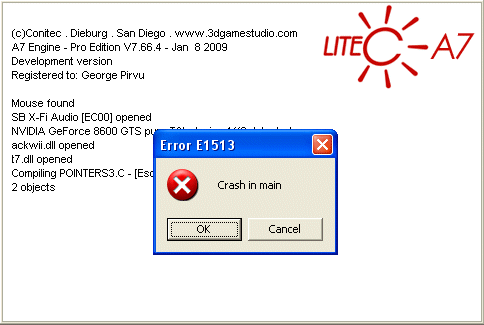It's obvious that something went wrong here... Let's examine the vector definition line carefully one more time (don't just read it, examine it!):   VECTOR* my_vector;   And now let's take a look at some of the lite-C pointer definitions from above:   BMAP* picture1_pcx = "picture1.pcx";   SOUND* effect2_wav = "effect2.wav";   STRING* hello_str = "Hello World!";   What is the significant difference between the vector pointer definition and these pointer definitions? Try to find the answer by yourself!   The answer is simple: the pointers that work fine are assigned to something: picture1_pcx points to picture1.pcx, effect2_wav points to effect2.wav and so on. Our vector pointer was created, but it wasn't pointing to anything, so trying to access its x component was leading (of course) to a crash.   We can assign our vector pointer to an existing vector or we can initialize it directly, just like we did it with the other pointers:   VECTOR* my_vector = {x = 1; y = 2; z = 3;} // this vector pointer definition works fine!   If we use something like this, we will be able to play with the vector, changing the value of its components inside a function, etc. Nevertheless, the best idea is to avoid using vector pointers whenever it is possible; we only have 64 available vector pointer slots, so their content could get erased if we use many vector calls. Use vector pointers only when you really need to pass one or more vectors to a function, just like below:   function do_something(VECTOR* some_vector) {        // do something with the values of the vector here        // .......................        // don't forget to return the result of the operation! }   In this case, we don't need to store the values of some_vector; we simply pass them to the function in order to get the needed result and we are done with them. Now that I look at the function above, I realize that a practical example would be beneficial, so let's open and run the pointers4.c script: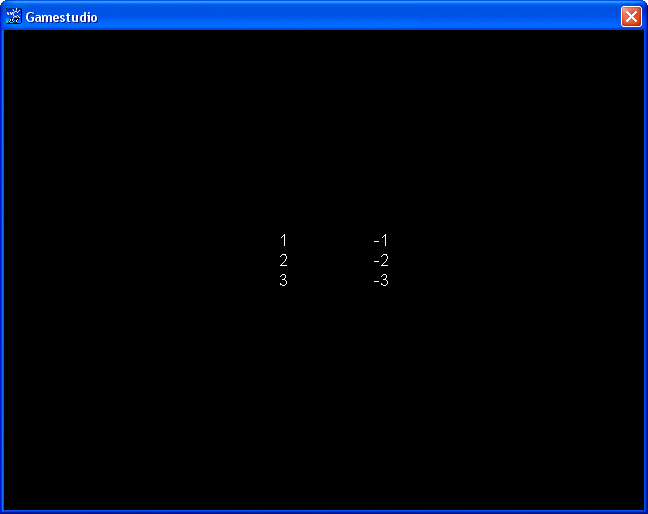VECTOR secret_code; VECTOR inv_result;   PANEL* time_pan = {        layer = 15;        digits (250, 200, 6, arial_font, 1, secret_code.x);                digits (250, 220, 6, arial_font, 1, secret_code.y);                digits (250, 240, 6, arial_font, 1, secret_code.z);                digits (350, 200, 6, arial_font, 1, inv_result.x);                digits (350, 220, 6, arial_font, 1, inv_result.y);                digits (350, 240, 6, arial_font, 1, inv_result.z);                flags = visible; }   function vec_invert(VECTOR* my_vector) {        inv_result.x = -my_vector.x;        inv_result.y = -my_vector.y;        inv_result.z = -my_vector.z;        return &inv_result; // we can't return more than a variable, so we return the address of inv_result }   function main() {        video_mode = 6; // create a program window of 640x480 pixels        vec_set(screen_color, vector(0, 0, 0)); // make the background color black        vec_set(secret_code.x, vector(1, 2, 3)); // let's set our secret code        vec_invert(secret_code); // and now let's invert it, so that nobody will be able to decipher it }   As you see, we are defining two regular vectors (not vector pointers); one of them will store our secret code, and the other one will store the encrypted code (the negative values of the secret code vector). We are using a panel with 6 digits instructions that display the x y z components for our 2 vectors. Function main sets our secret code to (1, 2, 3) and then calls function vec_invert:   function vec_invert(VECTOR* my_vector) {        inv_result.x = -my_vector.x;        inv_result.y = -my_vector.y;        inv_result.z = -my_vector.z;        return &inv_result; // we can't return more than a variable, so we return the address of inv_result }   This function uses a vector pointer as its parameter; the code inverts each component of the vector and returns the result. The bad news is that a function can't return more than a value, so it can't return all the components for our vector. Fear not, though, because there's a way: we can return the address of our vector and get all its components; this is exactly what the "return &inv_result;" line of code does.   As a conclusion, if you need a permanent vector, don't use a pointer to a vector, but a REAL vector definition such as this one:   VECTOR my_position;   You can use global or local vectors without worrying about any potential errors; they are nothing more than variables, so they don't have to be initialized or to point to something. Use a vector pointer only when you need to pass the values of a vector to a function.   Back to our pointer discussion: we can, of course, define pointers without assigning them to an object, as long as we don't use them until they get to point to something:   ENTITY* my_robot; // valid pointer definition   PANEL* winner_pan; // valid pointer definition   However, it would be a huge mistake to repeat the VECTOR* error and try to access my_robot.x or winner_pan.alpha before telling the engine which is the entity or the panel that's pointed by our pointer. Open up and run pointers5.c; you should see another error message: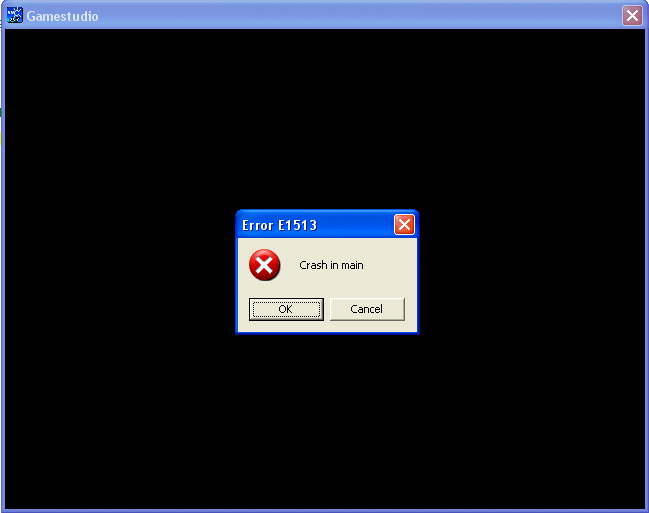Let's examine the code carefully:   #include #include   ENTITY* r2_robot;   action robot1() {        r2_robot = my; // now the r2_robot pointer is assigned to the entity that has this action attached to it }   function main() {        video_mode = 6; // create a program window of 640x480 pixels        level_load("test.wmb");        r2_robot.z += 100; }   Isn't it amazing that we have managed to produce a bug with so little code? Let's see what went wrong. First of all, we are defining an entity pointer named r2_robot - that's fine. Then, we have an action that assigns our entity pointer to the model that has action robot1( ) attached to it in Wed - our robot! Finally, function main loads the level and tell the robot to move 100 quants upwards. Everything appears to be working fine, so where's the error? Try to figure this one by yourself without peeking at the solution below.The pointer definition and assignment are ok, but we're having a timing bug: the "level_load" instruction needs some time in order to load the entire level from the hard drive, so r2_robot.z += 100; errors out because the robot model isn't loaded yet. The solution is simple: insert a "wait" instruction before accessing the robot:   function main() {        video_mode = 6; // create a program window of 640x480 pixels        level_load("test.wmb");        wait (3); // wait until the level and the entities from the level are loaded        r2_robot.z += 100; }   An even better solution is to make sure that the robot pointer is valid before accessing it, just like this:   function main() {        video_mode = 6; // create a program window of 640x480 pixels        level_load("test.wmb");        while (!r2_robot) {wait (1);} // wait until the robot pointer becomes valid (the robot model is loaded in the level)        r2_robot.z += 100; }   This was a long workshop, but I hope that you are much more comfortable with the lite-C pointers now. Next month we will deal with lite-C structs, special objects that can contain variables, pointers or even other structs inside them.